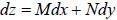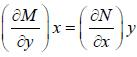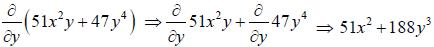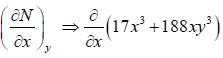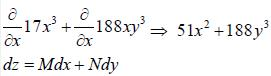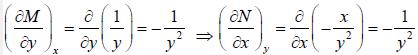Courses

# Chemical Thermodynamics

## 20 Questions MCQ Test GATE Chemistry Mock Test Series 2020 | Chemical Thermodynamics

Description
This mock test of Chemical Thermodynamics for Chemistry helps you for every Chemistry entrance exam. This contains 20 Multiple Choice Questions for Chemistry Chemical Thermodynamics (mcq) to study with solutions a complete question bank. The solved questions answers in this Chemical Thermodynamics quiz give you a good mix of easy questions and tough questions. Chemistry students definitely take this Chemical Thermodynamics exercise for a better result in the exam. You can find other Chemical Thermodynamics extra questions, long questions & short questions for Chemistry on EduRev as well by searching above.
QUESTION: 1

### For which set of ΔH• and ΔS- will the reaction be spontaneous only at high temperature

Solution:

At high temperature, ΔG = ΔH - TΔS
Only, ΔH = +ve , ΔS = +ve, T = high
Reaction spontaneous, ΔG = -ve

*Answer can only contain numeric values
QUESTION: 2

### The final temperature of one mole of gas at 200 atm an d 19°C as it is forced through a porous plug to a final pressure of 0.95 atm is _______________ K( μJT  = 0.150 k atm) (Round off to two decimal places).

Solution: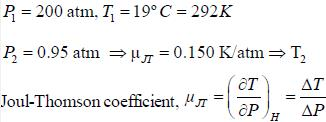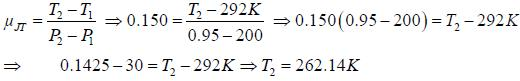QUESTION: 3

###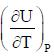is equal to

Solution: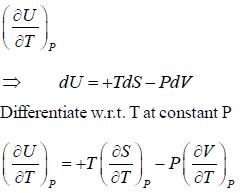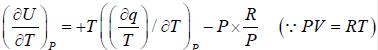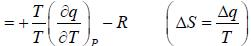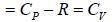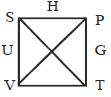*Answer can only contain numeric values
QUESTION: 4

A quantity of CH4 (g) at 298K is compressed reversibly and adiabatically from 50.0 bar to 200 bar. Assuming ideal behaviour. The value of final temperature of CH4 (g).  (Take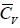= 3R ) ______________ (answer should be an integer).

Solution: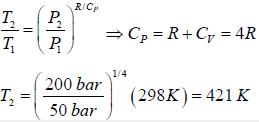QUESTION: 5

Hie change in entropy, when temperature is lower down to 1/8 of initial temperature at constant volume is

Solution: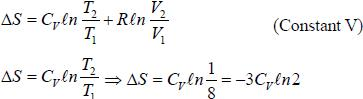*Answer can only contain numeric values
QUESTION: 6

The change in enthalpy when 5 mole of liquid benzene transforms to the vapour state at its boiling temperature(90°C) and at 1 bar pressure is (ΔSV = 87J K -mol) _____________________KJ. (Round off to two decimal places).

Solution: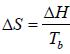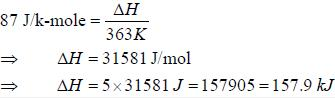QUESTION: 7

A steam engine operates between 400K and 300K under higher pressure. The minimum amount of heat that must be with drawn from the hot reservoir to obtain 1000 J of work is

Solution: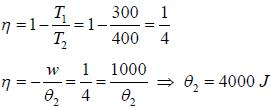QUESTION: 8

For a vander Waal gas of n mole, the expression for ΔH is

Solution: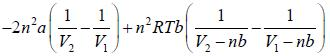QUESTION: 9

The change in entropy if one mole of S02(g) at 300K and 1.00 bar is heated to 1000K and its pressure is decreased to 0.010 bar. The molar heat InCorrect capacity of SC2(g) to be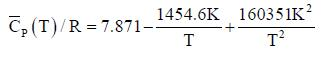Solution: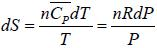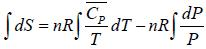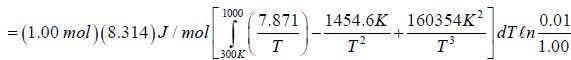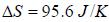QUESTION: 10

The change in entropy for the following transformation is respectively (+ indicates increases. - indicates decreases and 0 indicates no change)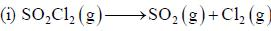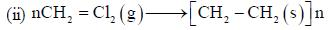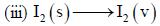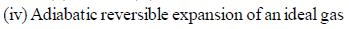Solution:

(i) Change in entropy = positive
(ii) Change in entropy = negative
(iii) Change in entropy = positive
(iv) Change in entropy = zero

QUESTION: 11

A gas obeys the equation P (Vm - b) = RT.  For this gas  b = 0.0391 dm3 mol-1 .The fugacity coefficient for the gas at 1000°C and 1000 atm is

Solution: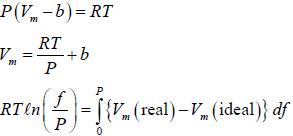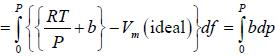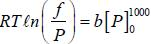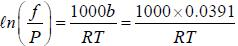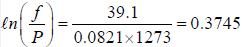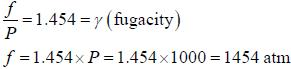QUESTION: 12

The correct relation for ΔU in van der Waal gas (in isothermal variation ) is

Solution: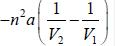QUESTION: 13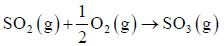is exothermic by 97030 J at 298K and 1 atm measured in a bomb calorimeter. The value of ΔH is

Solution: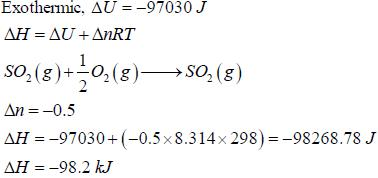*Answer can only contain numeric values
QUESTION: 14

The ΔGmix  when 10 moles of Ne get mixed with 20 moles of equimolar mixture of He and Ne is ____________kJ.(Round off to one decimal place)

Solution: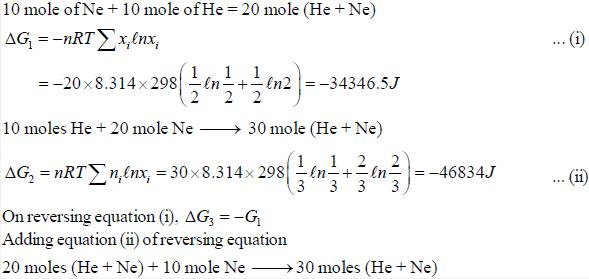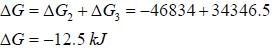*Answer can only contain numeric values
QUESTION: 15

A system filled with 0.505 mol of gas contracts reversibly from 1L to 0.10 L at constant temperature at 5°C. It simultaneously loses 1270 J of heat. The ΔU is____________J. (Round off to nearest integer))

Solution:

q = -1270J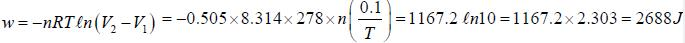Even through process is isothermal but overall ΔU change is not zero. So. applying first law
ΔU = q + w = -1270 + 2688 = 14187

*Answer can only contain numeric values
QUESTION: 16

The molar heat capacity at constant pressure of  H2O (g) at 1.00 atm is given by
Cp =30.54 + 10.3 x 10-2 T
The change in entropy when 2.0 mole of water at 1.00 atm. The temperature of H2O(g) is increased from 300K to 1000K is____________J K. (Round off to two decimal places)

Solution: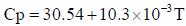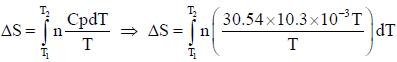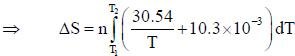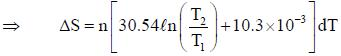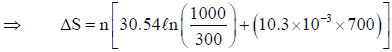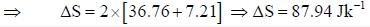QUESTION: 17

The molecular partition function of a system is given by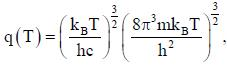where the symbols have their usual meanings. The heat capacity at constant volume for this system is

Solution: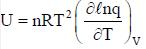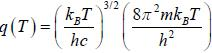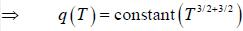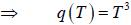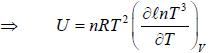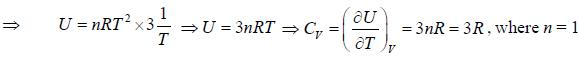QUESTION: 18

1 mole of N2, 2 mole of O2 and 1 mole of CO2 were mixed at 300K. The entropy of mixing is

Solution: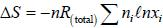'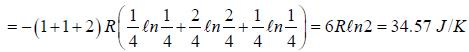QUESTION: 19

One mole of an ideal ga s Cv = 1.5 R at temperature 40 0K is com pressed from 2 atm to 3 atm by a reversible isothermal path. Subsequantly, it is expanded back to 2 atm by a reversible adiabatic path. The value of final state in litre is

Solution: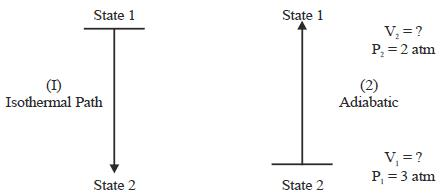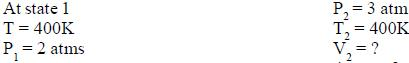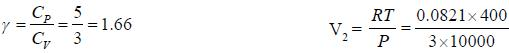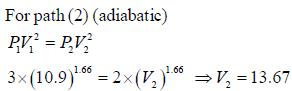QUESTION: 20

Choose the correct option in the favour of exact differential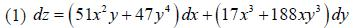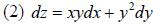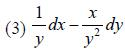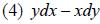Solution: